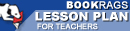Lesson Plans

# The Logic of Scientific Discovery Quiz | Eight Week Quiz C

This set of Lesson Plans consists of approximately 102 pages of tests, essay questions, lessons, and other teaching materials.
 View a FREE sampleName: _________________________ Period: ___________________

This quiz consists of 5 multiple choice and 5 short answer questions through Part II: Chapter 4, Falsifiability.

## Multiple Choice Questions

1. What should the rules of epistemology adhere to?
(a) Psychology.
(b) Logic.
(c) Demarcation.
(d) Scientific theory.

2. What depends on both universal and singular statements?
(a) Causality.
(b) Abstract thought.
(c) Logical factors.
(d) Correlations.

3. What does Popper say about positivism?
(a) It is too narrow, limited, and misguided.
(b) It is a clear way of thinking.
(c) It is based on invalid assumptions.
(d) It is based on scientific data.

4. What is the theory of theories?
(a) Existential statements.
(b) Causality.
(c) The logic of science.
(d) System of theories.

5. To what does Popper equate critical thinking?
(a) Common sense.
(b) Inductive thinking.
(c) Rational attitude.
(d) Deductive thinking.

1. What is one characteristic of the relationship between theory and classes of statements that must be present?

2. What happens if there is on falsifiable information?

3. What is not to be expected of methodology?

4. What does Popper consider existential statements to be?

5. What is diametrically opposed to the critical attitude required of a scientist?

 This section contains 175 words (approx. 1 page at 300 words per page) View a FREE sample